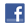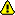Get 1 free lesson per week // Add a new lessonNew account
Millions of accounts created on our sites.

• Home
• Print
• Guestbook
• Report a bug

•Get a free English lesson every week!

Partners:
- Our other sites

Learn English > English lessons and exercises > English test #18778: Numbers> Other English exercises on the same topic: Numbers [Change theme] > Similar tests: - Cardinal and ordinal numbers - Fractions and percentages - ESL-Numbers from 1 to 10 - Geometric shapes - Vocabulary: mathematics - Geometry-Vocabulary - Idioms: numbers - Numbers and dates... (Level 1) > Double-click on words you don't understand

# Numbers

Numbers

Cardinal numbers

379 = three hundred and seventy-nine

2,860 = two thousand eight hundred and sixty

5,084 = five thousand and eighty-four

470,000 = four hundred and seventy thousand

2,550,000 = two million, five hundred and fifty thousand

3,000,000,000 = three billion

Note : there is no plural 's' after hundred, thousand, million, and billion when they are part of a number. On their own, they can be plural, e.g. thousands of people; millions of insects.

Ordinal numbers and dates

One of the problems with dates is that we write them and say them in a different way:

we write 4(th) January, but we say the fourth of January or January the fourth.

we write 21(st) May , but we say the twenty-first of May or May the twenty-first.

1905 = nineteen hundred and five or nineteen oh five.

1997 = nineteen ninety-seven.

2007 = two thousand and seven or two thousand oh seven.

Fractions and decimals

1 1/4 = one and a quarter

1 1/2 = one and a half

1 3/4 = one and three quarters

1 1/3 = one and a third

1.5 = one point five

1.46 = one point four six

Percentages

26% = twenty-six per cent

More than 50% is the majority; less than 50% is the minority

Arithmetic

There are four basic processes for working out (= calculating) a problem:

+ = addition         e.g. 6+4 = 10  (six plus/and four equals/is ten)

- = subtraction     e.g. 6-4 = 2 (six minus four equals/is two)

x = multiplication  e.g. 6x4 = 24 (six times/multiplied by four equals/is twenty-four)

/ = division           e.g. 4/2 = 2 (four divided by two equals/is two)

Saying '0'

This can be spoken in different ways in different contexts.

telephone numbers 603 744 = six oh three, seven double four

mathematics: 0.7 = nought point seven

6.02 = six point oh two

temperature: -10 degrees = ten degrees below zero/minus ten degrees

football: 2-0 = two nil

tennis: 40-0 = forty love

Talking numbers

Here are several useful words and expressions connected with numbers:

The streets have got odd numbers (e.g. 3,5,7) on the left and even numbers (e.g. 4,6,8) on the right.

I got 16 out of 20 in our last test.

------Twitter Share
English exercise "Numbers" created by anonyme with The test builder.Please log in to save your progress.

462

2 1/2

2,345

6.75

0.25

3 1/3

1,250,000

10.04

52%

September, 10th = the

July, 3rd = the

602 8477 [phone number]

1903 [date]

1876 [date]

2-0 [football match]

1 3/4

3,000,000,000

2,000,000

End of the free exercise to learn English: Numbers
A free English exercise to learn English.
Other English exercises on the same topic : Numbers | All our lessons and exercises